The Dot Product

Vector dot product is  also called a scalar product because the product of vectors gives a scalar quantity. Sometimes, a dot product is also named as an inner product. In vector algebra, dot product is an operation applied on vectors

The Scalar product or dot product is commutative. When two vectors are operated under a dot product, the answer is only a number. A brief explanation on dot products is given below.

When two vectors are combined under addition or subtraction, the result is a vector. When two vectors are combined using the dot product, the result is a scalar. For this reason, the dot product is often called the scalar product. It may also be called the inner product.

Dot product of two vector

If we have two vectors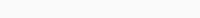then the dot product or scalar product between them is defined as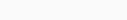## Formula for vectors Dot Product

Let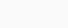be two non zero vectors. Then, the scalar product is denoted by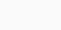and is defined as the scalar## Dot Product of Two Parallel Vectors

If two vectors have the same direction or two vectors are parallel to each other, then the dot product of two vectors is the product of their magnitude.

Here, θ = 0 degree

so, cos 0 = 1

Therefore,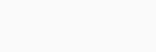## Dot Product of Opposite Vectors

If the two vectors are opposite in direction, then θ=π

Here,

cos⁡π=−1

Now,Let us know about some properties of Dot product.

Let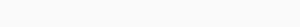• Commutative Property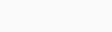• Distributive Property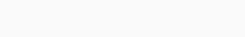• Associative Property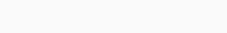• property of magnitude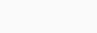Let us solve an example using  S2 for better understanding.

Q: Determine $$x .y$$ given $$x = \begin{bmatrix}1\\2\\3\\4\end{bmatrix}$$ and $$y = \begin{bmatrix}4\\5\\6\\7\end{bmatrix}$$

				
%use s2
// define vectors x and y
var x = DenseVector(arrayOf(1.0, 2.0, 3.0, 4.0))
var y = DenseVector(arrayOf(4.0, 5.0, 6.0, 7.0))

// P = x.y
val P = x.multiply(y)
println(P)


[4.000000, 10.000000, 18.000000, 28.000000]

Geometrical Connection:

The dot product $$x . y$$ can also be calculated with the help of the angle $$\theta$$ between these two vectors as follows:

$$x . y =\left|x\right|\left|y\right|cos \theta$$

Let us find the dot product of the vectors in the figure below:The Transpose

The transpose of a matrix is one of the most commonly used methods in matrix transformation. For a given matrix, the transpose of a matrix is obtained by interchanging rows into columns or columns to rows. In this article, we are going to learn the definition of the transpose of a matrix, steps to find the transpose of a matrix, properties and examples with a complete explanation.

The transpose of a matrix is found by interchanging its rows into columns or columns into rows. The transpose of the matrix is denoted by using the letter “T” in the superscript of the given matrix. For example, if “A” is the given matrix, then the transpose of the matrix is represented by A’ or AT.

Definition: If $$A$$ is an $$m \times n$$ matrix, then its transpose $$A^T$$ is defined to be the matrix with $$n$$ rows whose $$i^{th}$$ row is equal to the $$i^{th}$$ column of $$A$$, for each $$i$$ from $$1$$ to $$n$$.

If $$A = \begin{bmatrix}1 & 2 & 3\\4 & 5 & 6\end{bmatrix}$$, then $$A^T = \begin{bmatrix}1 & 4\\2 & 5\\3 & 6\end{bmatrix}$$

Let us implement the same using S2.

				
%use s2
// define a matrix
var A = DenseMatrix(arrayOf(
doubleArrayOf(1.0, 2.0, 3.0),
doubleArrayOf(4.0, 5.0, 6.0)))
println(A)

// B = Tranpose of A
val B = A.t()
println("\n\nTranspose of A:\n")
println(B)


2x3
[,1] [,2] [,3]
[1,] 1.000000, 2.000000, 3.000000,
[2,] 4.000000, 5.000000, 6.000000,

Transpose of A:
3x2
[,1] [,2]
[1,] 1.000000, 4.000000,
[2,] 2.000000, 5.000000,
[3,] 3.000000, 6.000000, 

Matrix

When we take only the real constants of a linear equation or a system of linear equations and make an array then it becomes a matrix. There are different types of matrix which are identified and defined based on the structure consisting of elements in different ways and existing in different positions. Writing the real constants of a linear equation or a system of linear equations in a rectangular array is a matrix. All the elements of a matrix are called entries [2,3].

Let the following system of linear equationsThen the matrix of the above system of linear equations isMatrix Properties

Now that we know what the transpose of a matrix is, let’s learn about various matrix properties and their illustrations in S2.

Symmetric Matrix

A symmetric matrix in linear algebra is a square matrix that remains unaltered when its transpose is calculated. That means, a matrix whose transpose is equal to the matrix itself, is called a symmetric matrix.A matrix in which its $$(i,j)^{th}$$ entry will be necessarily equal to its $$(j,i)^{th}$$ entry is known as a Symmetric Matrix.

In other words, if $$A$$ is an $$n \times n$$ matrix satisfying the equation $$A = A^T$$, we say that $$A$$ is symmetric.

				
%use s2
// define a matrix
var A = DenseMatrix(arrayOf(
doubleArrayOf(1.0, 2.0, 3.0),
doubleArrayOf(2.0, 4.0, 5.0),
doubleArrayOf(3.0, 5.0, 6.0)))
println(A)

print("\n")
//prints 'true' if A is symmetric, else prints 'false'
val precision = 1e-15
println(MatrixPropertyUtils.isSymmetric(A,precision))


3x3
[,1] [,2] [,3]
[1,] 1.000000, 2.000000, 3.000000,
[2,] 2.000000, 4.000000, 5.000000,
[3,] 3.000000, 5.000000, 6.000000,

true

Skew-Symmetric Matrix

A skew symmetric matrix is defined as the square matrix that is equal to the negative of its transpose matrix. For any square matrix, A, the transpose matrix is given as AT. A skew-symmetric or antisymmetric matrix A can therefore be represented as, A = -ATIf $$A$$ is an $$n \times n$$ matrix satisfying the equation $$A^T = -A$$, we say $$A$$ is Skew-Symmetric.

				
%use s2
// define a matrix
var A = DenseMatrix(arrayOf(
doubleArrayOf(0.0, -6.0, 4.0),
doubleArrayOf(6.0, 0.0, -5.0),
doubleArrayOf(-4.0, 5.0, 0.0)))
println(A)

print("\n")
//prints 'true' if A is skew-symmetric, else prints 'false'
val precision = 1e-15
println(MatrixPropertyUtils.isSkewSymmetric(A,precision))


3x3
[,1] [,2] [,3]
[1,] 0.000000, -6.000000, 4.000000,
[2,] 6.000000, 0.000000, -5.000000,
[3,] -4.000000, 5.000000, 0.000000,

true

Idempotent Matrix

An idempotent matrix is a matrix which, when multiplied by itself, yields itself. That is, the matrix is idempotent if and only if . For this product to be defined, it must necessarily be a square matrix. Viewed this way, idempotent matrices are idempotent elements of matrix rings.

If $$A$$ is an $$n \times n$$ matrix satisfying the equation $$A = A*A$$ or $$A = A^2$$, we say $$A$$ is Idempotent.%use s2
// define a matrix
var A = DenseMatrix(arrayOf(
doubleArrayOf(2.0, -2.0, -4.0),
doubleArrayOf(-1.0, 3.0, 4.0),
doubleArrayOf(1.0, -2.0, -3.0)))
println(A)

//prints 'true' if A is idempotent, else prints 'false'
val precision = 6.0
println("\n")
println(MatrixPropertyUtils.isIdempotent(A,precision))


3x3
[,1] [,2] [,3]
[1,] 2.000000, -2.000000, -4.000000,
[2,] -1.000000, 3.000000, 4.000000,
[3,] 1.000000, -2.000000, -3.000000,

true

Orthogonal Matrix

A square matrix with real numbers or elements is said to be an orthogonal matrix if its transpose is equal to its inverse matrix. Or we can say when the product of a square matrix and its transpose gives an identity matrix, then the square matrix is known as an orthogonal matrix1If $$A$$ is an $$n \times n$$ matrix satisfying the equation $$A.A^T = A^T.A = I$$ where $$I$$ is an Identity Matrix of order $$n$$, we say $$A$$ is Orthogonal.

				
%use s2
// define a matrix
var A = DenseMatrix(arrayOf(
doubleArrayOf(1.0, 0.0, 0.0),
doubleArrayOf(0.0, 0.0, -1.0),
doubleArrayOf(0.0, -1.0, 0.0)))
println(A)

//prints 'true' if A is orthogonal, else prints 'false'
val precision = 1e-15
println("\n")
println(MatrixPropertyUtils.isOrthogonal(A,precision))


3x3
[,1] [,2] [,3]
[1,] 1.000000, 0.000000, 0.000000,
[2,] 0.000000, 0.000000, -1.000000,
[3,] 0.000000, -1.000000, 0.000000,

true